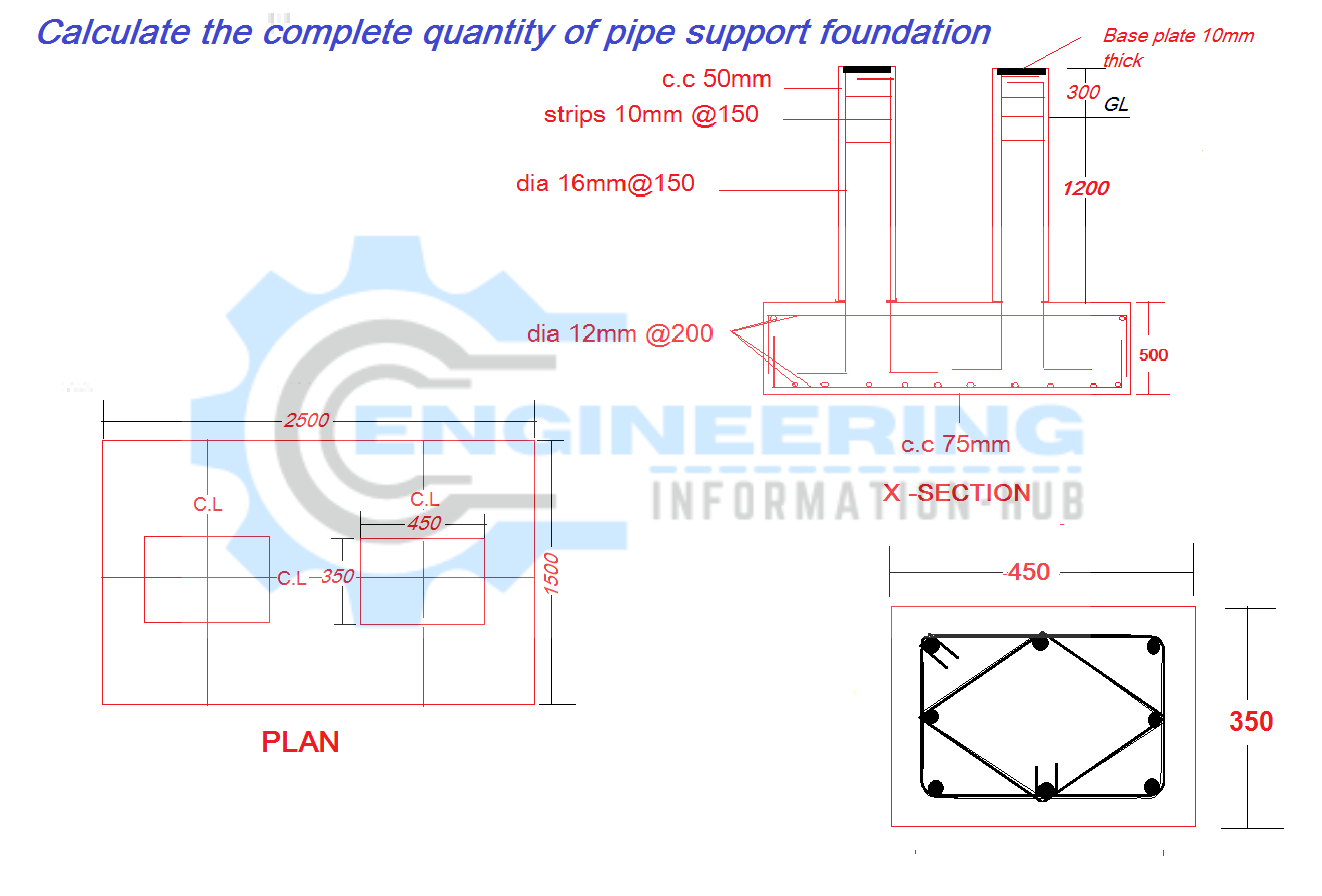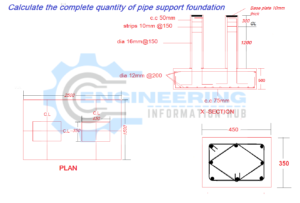Bar Bending Schedule

# Calculate the Complete Quantity of Pipe Support Foundation

## Calculate the Complete Quantity of Pipe Support Foundation

calculate the volume of concrete and rebar excavation backfilling etcfooting and pedestal. quantity of backfilling materials. a number of the base plate.

I have some given data

I have one pipe support drawing they have mentioned in all detail.

length of pipe support foundation =2.5m

width of pipe support foundation=1.5m

height of footing =0.500m

Total height of pedestal =1.5m

above ground =0.300m

length of pedestal =0.450m

width of pedestal =0.350m

Now we calculate the excavation of pipe support

total excavation of pipe support foundation = L×W×H

total excavation of pipe support foundation =2×1.5×1.7=5.1m³

this is the exact excavation of the foundation but for working space, we will do 600mm all side extra excavation. and 100mm for lean concrete. so its means the excavation is

total excavation=3.2×2.7×1.8=15.552m³

### How To Calculate The Bar Bending Schedule for Lintel Beam

Now lean concrete

lean concrete is all side 100mm extra and the thickness of lean concrete is 100mm

solean concrete =2.2×1.7×0.100=0.374m³

Now concrete for the footing

concrete for footing =L×B×H

concrete for footing =2×1.5×0.500=1.5m³

now concrete for pedestal or column

concrere for pedestal or column= L×B×H

concrere for pedestal or column=0.450×0.350×1.5=0.236m³

this concrete is for one pedestal but we have 2 pedestals or columns for pipe support

so total concrete for two pedestals =0.236×2=0.472m³

now we calculate the quantity of rebar for footing

footing rebar

dia of the long bar =12mm

spacing of footing =200mm

dia of short  bar=12mm

Now calculate the footing long bar

cutting length of long bar= total length -2(C.C)+2 bends

cutting length of long bar=2000-2(75)+2(19d)=2000-150+2(228)=1394mm=1.394m

Nos of long bar = width of footing/spacing+1

Nos of long bar =1500/200+1=8.5=9bar

total cutting length of long bar =nos of bar×length of one bar

total cutting length of long bar =9×1.394=12.546m

Now

cutting length of short bar=widht of footing-2C.C+2bends

cutting length of short bar=1500-2(75)+2(19d)

cutting length of short bar=1500-150+2(19×12)

cutting length of short bar=1500-150+456=1806mm=1.806m

Nos of short bar =total length/spacing+1

Nos of short bar =2000/200+1=11bar

total cutting length of short bar=nos of bar×length of one bar

total cutting length of short bar=11×1.806=19.866m

Now we calculate the weight of the short and long bars at one time because the dia between the short and long bars is the same so that why.

the total length of long and short bar =length of short of bar+length of the long bar

the total length of long and short bar =12.546+19.866=32.412m

weight of long and short bar =d²/162.25×total length

weight of long and short bar =12²/162.25×32.412=28.766kg=29kg

Now calculate the vertical bar

dia of vertical bar=16mm

height of pedestal or column=1.5m

length of pedestal=450mm

width of pedestal=350mm

cutting length of vertical bar=total height of pipe support foundation-bottom c.c -2dia of the bottom bar -top c.c +bottom bends+top bends

cutting length of vertical bar=2000-75-2(12)-50+(18d)+10d

cutting length of vertical bar=2000-75-24-50+(18×16)+10×16

cutting length of vertical bar=2000-75-24-50+288+160=2.299m

we have 8 nos of the vertical bar

we have 2 pedestals =8×2=16 nos

total cutting length of vertical bar =nos of bar×length of one bar

total cutting length of vertical bar =16×2.299=36.784m

weight of vertical bar=d²/162.25×total length

weight of vertical bar=16²/162.25×36.784=58.038=59kg

now

we calculate the stirrups length and nos of stirrups

we have two types of stirrups one is a rectangle shape and one is a diamond shape

Now 1st rectangle stirrups

Nos of rectangle stirrups =total height of pedestal/spacing+1

Nos of rectangle stirrups =1500/150+1=11nos

we have 2 column=11×2=22nos

cutting length of stirrups=2 sides 350+2 sides 250+2bends

cutting length of stirrups=2(350)+2(250)+2(9d)

cutting length of stirrups=700+500+2(9×10)

cutting length of stirrups=1200+180=1380mm=1.380m

the total length of stirrups =nos of stirrups×length of one stirrup

the total length of stirrups =22×1.380=30.360m

Now calculate the diamond stirrups

no of diamond stirrups=nos of rectangle stirrups

22=22

cutting length of stirrups=4 sides of diamond stirrups +2bends

cutting length of stirrups=4(215)+2(9d)=860+2(9×10)=1.040m

total cutting length of stirrups=nos of stirrups×length of one stirrup

total cutting length of stirrups=22×1.040=22.88m

Now calculate the weight of both stirrups

dia of stirrups =10mm

the total length of stirrups=length of rectangle +length of diamond

total length of both stirrups=30.360+22.08=52.44m

weight of diamond stirrup =d²/162.25×total length

weight of diamond stirrups =10²/162.25×52.44=32.32=33kg

now we calculate the backfilling

backfilling of pipe support foundation up to ground level.

Quantity of backfilling=quantity of total excavation – Quantity of total concrete footing, pedestals, and lean concrete

total concrete=footing and pedestal concrete+lean concrete

total concrete=2.346+0.374=2.720m³

Quantity of backfilling=15.552-2.720=12.832m³

Nos of base plate =2

### Raja Numan

Hi, My name is Engr. Raja Numan author of Engineering Information Hub and I am a Civil Engineer by Profession and I've specialized in the field of Quantity Surveying, Land Surveying as QC Engineer in national and multinational companies of Pakistan & Saudi Arabia.